# The Four Types of Estimable Functions

### Type III and IV SS and Estimable Functions

Subsections:

When an effect is contained in another effect, the Type II hypotheses for that effect are dependent on the cell frequencies. The philosophy behind both the Type III and Type IV hypotheses is that the hypotheses tested for any given effect should be the same for all designs with the same general form of estimable functions.

To demonstrate this concept, recall the hypotheses being tested by the Type II SS in the balancedfactorial shown in Table 15.6. Those hypotheses are precisely the ones that the Type III and Type IV hypotheses employ for allfactorials that have at least one observation per cell. The Type III and Type IV hypotheses for a design without missing cells usually differ from the hypothesis employed for the same design with missing cells since the general form of estimable functions usually differs.

Many SAS/STAT procedures can perform tests of Type III hypotheses, but only PROC GLM offers Type IV tests as well.

#### Type III Estimable Functions

Type III hypotheses are constructed by working directly with the general form of estimable functions. The following steps are used to construct a hypothesis for an effect F1:

1. For every effect in the model except F1 and those effects that contain F1, equate the coefficients in the general form of estimable functions to zero.

If F1 is not contained in any other effect, this step defines the Type III hypothesis (as well as the Type II and Type IV hypotheses). If F1 is contained in other effects, go on to step 2. (See the section Type II SS and Estimable Functions for a definition of when effect F1 is contained in another effect.)

2. If necessary, equate new symbols to compound expressions in the F1 block in order to obtain the simplest form for the F1 coefficients.

3. Equate all symbolic coefficients outside the F1 block to a linear function of the symbols in the F1 block in order to make the F1 hypothesis orthogonal to hypotheses associated with effects that contain F1.

By once again observing the Type II hypotheses being tested in the balancedfactorial, it is possible to verify that the A and A * B hypotheses are orthogonal and also that the B and A * B hypotheses are orthogonal. This principle of orthogonality between an effect and any effect that contains it holds for all balanced designs. Thus, construction of Type III hypotheses for any design is a logical extension of a process that is used for balanced designs.

The Type III hypotheses are precisely the hypotheses being tested by programs that reparameterize using the usual assumptions (for example, constraining all parameters for an effect to sum to zero). When no missing cells exist in a factorial model, Type III SS coincide with Yates’ weighted squares-of-means technique. When cells are missing in factorial models, the Type III SS coincide with those discussed in Harvey (1960) and Henderson (1953).

The following discussion illustrates the construction of Type III estimable functions for afactorial with no missing cells.

To obtain the A * B interaction hypothesis, start with the general form and equate the coefficients for effects, A, and B to zero, as shown in Table 15.8.

Table 15.8: Type III Hypothesis for A * B Interaction

Effect

General Form

L1 = L2 = L4 = 0L1

0

A1

L2

0

A2

L1L2

0

B1

L4

0

B2

L1L4

0

AB11

L6

L6

AB12

L2L6

L6

AB21

L4L6

L6

AB22

L1L2L4 + L6

L6

The last column in Table 15.8 represents the form of the MRH for A * B.

To obtain the Type III hypothesis for A, first start with the general form and equate the coefficients for effectsand B to zero (let L1 = L4 = 0). Next let L6 = KL2, and find the value of K that makes the A hypothesis orthogonal to the A * B hypothesis. In this case, K = 0.5. Each of these steps is shown in Table 15.9.

In Table 15.9, the fourth column (under L6 = KL2) represents the form of all estimable functions not involving, B1, or B2. The prime difference between the Type II and Type III hypotheses for A is the way K is determined. Type II chooses K as a function of the cell frequencies, whereas Type III chooses K such that the estimable functions for A are orthogonal to the estimable functions for A * B.

Table 15.9: Type III Hypothesis for A

Effect

General Form

L1 = L4 = 0

L6 = KL2

K= 0.5L1

0

0

0

A1

L2

L2

L2

L2

A2

L1L2

L2

L2

L2

B1

L4

0

0

0

B2

L1L4

0

0

0

AB11

L6

L6

KL2

0.5L2

AB12

L2L6

L2L6

(1 – K)L2

0.5L2

AB21

L4L6

L6

KL2

–0.5L2

AB22

L1L2L4 + L6

L2 + L6

–(1 – K)L2

–0.5L2

An example of Type III estimable functions in a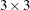factorial with unequal cell frequencies and missing diagonals is given in Table 15.10 (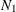through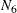represent the nonzero cell frequencies).

Table 15.10:Factorial Design with Unequal Cell Frequencies and Missing Diagonals

 B 1 2 3 1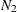A 2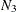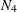3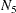For any nonzero values ofthrough, the Type III estimable functions for each effect are shown in Table 15.11.

Table 15.11: Type III Estimable Functions forFactorial Design with Unequal Cell Frequencies and Missing Diagonals

Effect

A

B

A * B0

0

0

A1

L2

0

0

A2

L3

0

0

A3

L2L3

0

0

B1

0

L5

0

B2

0

L6

0

B3

0

L5L6

0

AB12

0.667L2 + 0.333L3

0.333L5 + 0.667L6

L8

AB13

0.333L2 – 0.333L3

–0.333L5 – 0.667L6

L8

AB21

0.333L2 + 0.667L3

0.667L5 + 0.333L6

L8

AB23

–0.333L2 + 0.333L3

–0.667L5 – 0.333L6

L8

AB31

–0.333L2 – 0.667L3

0.333L5 – 0.333L6

L8

AB32

–0.667L2 – 0.333L3

–0.333L5 + 0.333L6

L8

#### Type IV Estimable Functions

By once again looking at the Type II hypotheses being tested in the balancedfactorial (see Table 15.6), you can see another characteristic of the hypotheses employed for balanced designs: the coefficients of lower-order effects are averaged across each higher-level effect involving the same subscripts. For example, in the A hypothesis, the coefficients of AB11 and AB12 are equal to one-half the coefficient of A1, and the coefficients of AB21 and AB22 are equal to one-half the coefficient of A2. With this in mind, the basic concept used to construct Type IV hypotheses is that the coefficients of any effect, say F1, are distributed equitably across higher-level effects that contain F1. When missing cells occur, this same general philosophy is adhered to, but care must be taken in the way the distributive concept is applied.

Construction of Type IV hypotheses begins as does the construction of the Type III hypotheses. That is, for an effect F1, equate to zero all coefficients in the general form that do not belong to F1 or to any other effect containing F1. If F1 is not contained in any other effect, then the Type IV hypothesis (and Type II and III) has been found. If F1 is contained in other effects, then simplify, if necessary, the coefficients associated with F1 so that they are all free coefficients or functions of other free coefficients in the F1 block.

To illustrate the method of resolving the free coefficients outside the F1 block, suppose that you are interested in the estimable functions for an effect A and that A is contained in AB, AC, and ABC. (In other words, the main effects in the model are A, B, and C.)

With missing cells, the coefficients of intermediate effects (here they are AB and AC) do not always have an equal distribution of the lower-order coefficients, so the coefficients of the highest-order effects are determined first (here it is ABC). Once the highest-order coefficients are determined, the coefficients of intermediate effects are automatically determined.

The following process is performed for each free coefficient of A in turn. The resulting symbolic vectors are then added together to give the Type IV estimable functions for A.

1. Select a free coefficient of A, and set all other free coefficients of A to zero.

2. If any of the levels of A have zero as a coefficient, equate all of the coefficients of higher-level effects involving that level of A to zero. This step alone usually resolves most of the free coefficients remaining.

3. Check to see if any higher-level coefficients are now zero when the coefficient of the associated level of A is not zero. If this situation occurs, the Type IV estimable functions for A are not unique.

4. For each level of A in turn, if the A coefficient for that level is nonzero, count the number of times that level occurs in the higher-level effect. Then equate each of the higher-level coefficients to the coefficient of that level of A divided by the count.

An example of afactorial with four missing cells (throughrepresent positive cell frequencies) is shown in Table 15.12.

Table 15.12:Factorial Design with Four Missing Cells

 B 1 2 3 1A 23The Type IV estimable functions are shown in Table 15.13.

Table 15.13: Type IV Estimable Functions forFactorial Design with Four Missing Cells

Effect

A

B

A * B0

0

0

A1

L3

0

0

A2

L3

0

0

A3

0

0

0

B1

0

L5

0

B2

0

L5

0

B3

0

0

0

AB11

–0.5L3

0.5L5

L8

AB12

–0.5L3

–0.5L5

L8

AB21

0.5L3

0.5L5

L8

AB22

0.5L3

–0.5L5

L8

AB33

0

0

0

#### A Comparison of Type III and Type IV Hypotheses

For the vast majority of designs, Type III and Type IV hypotheses for a given effect are the same. Specifically, they are the same for any effect F1 that is not contained in other effects for any design (with or without missing cells). For factorial designs with no missing cells, the Type III and Type IV hypotheses coincide for all effects. When there are missing cells, the hypotheses can differ. By using the GLM procedure, you can study the differences in the hypotheses and then decide on the appropriateness of the hypotheses for a particular model.

The Type III hypotheses for three-factor and higher completely nested designs with unequal Ns in the lowest level differ from the Type II hypotheses; however, the Type IV hypotheses do correspond to the Type II hypotheses in this case.

When missing cells occur in a design, the Type IV hypotheses might not be unique. If this occurs in PROC GLM, you are notified, and you might need to consider defining your own specific comparisons.